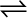7.25 Does the number of moles of reaction products increase, decrease or remain the same when each of the following equilibria is subjected to a decrease in pressure by increasing the volume?

(a) PCl5 (g)PCl3 (g) + Cl2 (g)

(b) CaO (s) + CO2 (g)CaCO3 (s)

(c) 3Fe (s) + 4H2O (g)Fe3O4 (s) + 4H2 (g)

(a) The number of moles of reaction products will increase. According to Le Chatelier’s principle, if pressure is decreased, then the equilibrium shifts in the direction in which the number of moles of gases is more. In the given reaction, the number of moles of gaseous products is more than that of gaseous reactants. Thus, the reaction will proceed in the forward direction. As a result, the number of moles of reaction products will increase.

(b) The number of moles of reaction products will decrease.

(c) The number of moles of reaction products remains the same.